## 【Day31】461. 汉明距离

#### 461. 汉明距离

• 0 ≤ x, y < 231.

输入: x = 1, y = 4

1   (0 0 0 1)
4   (0 1 0 0)
↑   ↑

class Solution {
public:
int lowbit(int x) {
return x & (-x);
}
int hammingDistance(int x, int y) {
int ret = x ^ y;
int ans = 0;
while (ret) {
ret -= lowbit(ret);
ans++;
}
return ans;
}
};

## 【Day32】477.汉明距离总和

#### 477. 汉明距离总和

输入: 4, 14, 2

HammingDistance(4, 14) + HammingDistance(4, 2) + HammingDistance(14, 2) = 2 + 2 + 2 = 6.

• 数组中元素的范围为从 0到 10^9。
• 数组的长度不超过 10^4。

class Solution {
public:
int totalHammingDistance(vector<int>& nums) {
int ans = 0;
for (int i = 0; i < 31; i++) {
int cnt[]  = {0, 0};
for (auto& num : nums) {
cnt[num & 1]++;
num >>= 1;
}
ans += cnt * cnt;
}
return ans;
}
};

## 【Day33】1074.元素和为目标值的子矩阵数量

#### 1074. 元素和为目标值的子矩阵数量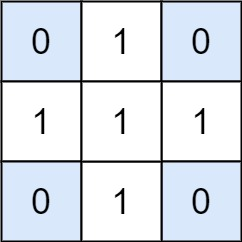输入：matrix = [[0,1,0],[1,1,1],[0,1,0]], target = 0

输入：matrix = [[1,-1],[-1,1]], target = 0

输入：matrix = [], target = 0

• 1 <= matrix.length <= 100
• 1 <= matrix.length <= 100
• -1000 <= matrix[i] <= 1000
• -10^8 <= target <= 10^8

#define HT_LENGTH 256

struct HashTable {
int keys[HT_LENGTH];
int vals[HT_LENGTH];

HashTable() {
this->clear();
}

void clear() {
keys = 0; vals = 1;
for (int i = 1;i < HT_LENGTH;i++) keys[i] = -1;
}

bool find(int key, int& ret) {
uint32_t ikey = (uint32_t)key % HT_LENGTH;
for (int i = 0;i < HT_LENGTH;i++) {
if (keys[ikey] == -1) return false;
if (keys[ikey] == key) {
ret = vals[ikey];
return true;
}
ikey ++;
ikey = ikey == HT_LENGTH ? 0 : ikey;
}
return false;
}

void inc(int key) {
uint32_t ikey = (uint32_t)key % HT_LENGTH;
for (int i = 0;i < HT_LENGTH;i++) {
if (keys[ikey] == -1) {
keys[ikey] = key;
vals[ikey] = 1;
return;
}
if (keys[ikey] == key) {
vals[ikey]++;
return;
}
ikey ++;
ikey = ikey == HT_LENGTH ? 0 : ikey;
}
}
};

class Solution {
private:
HashTable ht;
public:
int numSubmatrixSumTarget(vector<vector<int>>& matrix, int target) {
int rprefix;
int m = matrix.size(), n = matrix.size();
int ret = 0;
for (int i = 0;i < m;i++) {
rprefix[i] = 0;
for (int j = 1;j <= n;j ++) {
rprefix[i][j] = rprefix[i][j - 1] + matrix[i][j - 1];
}
}

for (int i = 1;i <= n;i++) {
for (int j = i;j <= n;j++) {
ht.clear();
int cprefix = 0, val;
for (int row = 0;row < m;row++) {
cprefix += rprefix[row][j] - rprefix[row][i - 1];
if (ht.find(cprefix - target, val)) ret += val;
ht.inc(cprefix);
}
}
}
return ret;
}
};

## 【Day34】231. 2 的幂

#### 231. 2 的幂

输入：n = 1

输入：n = 16

输入：n = 3

输入：n = 4

输入：n = 5

• -231 <= n <= 231 - 1

• n 二进制最高位为 11，其余所有位为 00；
• n−1 二进制最高位为 00，其余所有位为 11；

class Solution {
public:
bool isPowerOfTwo(int n) {
return n > 0 && (n & (n - 1)) == 0;
}
};

## 【Day35】342. 4的幂

#### 342. 4的幂

输入：n = 16

输入：n = 5

输入：n = 1

• -231 <= n <= 231 - 1

0x55555555的  二进制是  01010101 01010101 01010101 01010101
class Solution {
public:
bool isPowerOfFour(int n) {
return n > 0 && (n & (n - 1)) == 0 && (n & 0x55555555) == n;
}
};

## 【Day36】LeetCode 1744

#### 1744. 你能在你最喜欢的那天吃到你最喜欢的糖果吗？

• 你从第 0 天开始吃糖果。
• 你在吃完 所有 第 i - 1 类糖果之前，不能 吃任何一颗第 i 类糖果。
• 在吃完所有糖果之前，你必须每天 至少 吃 一颗 糖果。

输入：candiesCount = [7,4,5,3,8], queries = [[0,2,2],[4,2,4],[2,13,1000000000]]

1- 在第 0 天吃 2 颗糖果(类型 0），第 1 天吃 2 颗糖果（类型 0），第 2 天你可以吃到类型 0 的糖果。
2- 每天你最多吃 4 颗糖果。即使第 0 天吃 4 颗糖果（类型 0），第 1 天吃 4 颗糖果（类型 0 和类型 1），你也没办法在第 2 天吃到类型 4 的糖果。换言之，你没法在每天吃 4 颗糖果的限制下在第 2 天吃到第 4 类糖果。
3- 如果你每天吃 1 颗糖果，你可以在第 13 天吃到类型 2 的糖果。

输入：candiesCount = [5,2,6,4,1], queries = [[3,1,2],[4,10,3],[3,10,100],[4,100,30],[1,3,1]]

• 1 <= candiesCount.length <= 105
• 1 <= candiesCount[i] <= 105
• 1 <= queries.length <= 105
• queries[i].length == 3
• 0 <= favoriteTypei < candiesCount.length
• 0 <= favoriteDayi <= 109
• 1 <= dailyCapi <= 109

class Solution {
public:
vector<long long> sum;
bool check(vector<int>& candiesCount, vector<int>& q) {
int favoriteType = q, favoriteDay = q, dailyCap = q;
// 第favoriteType类型的前缀和
int l = sum[favoriteType + 1];
// 每天吃一颗糖
if (favoriteDay + 1 > sum[favoriteType + 1]) return false;
// 吃最多糖
if (sum[favoriteType] >= 1ll*(favoriteDay + 1) * dailyCap) return false;
return true;
}
vector<bool> canEat(vector<int>& candiesCount, vector<vector<int>>& queries) {
int n = candiesCount.size();
sum = vector<long long>(n + 1, 0);
for (int i = 1; i <= n; i++) {
sum[i] = sum[i - 1] + candiesCount[i - 1];
}

vector<bool> ans;
for (auto q : queries)
ans.push_back(check(candiesCount, q));
return ans;
}
};

## 【Day37】523.连续的子数组和

#### 523. 连续的子数组和

• 子数组大小 至少为 2 ，且
• 子数组元素总和为 k 的倍数。

输入：nums = [23,2,4,6,7], k = 6

输入：nums = [23,2,6,4,7], k = 6

42 是 6 的倍数，因为 42 = 7 * 6 且 7 是一个整数。

输入：nums = [23,2,6,4,7], k = 13

• 1 <= nums.length <= 105
• 0 <= nums[i] <= 109
• 0 <= sum(nums[i]) <= 231 - 1
• 1 <= k <= 231 - 1

class Solution {
public:
bool checkSubarraySum(vector<int>& nums, int k) {
int sum = 0;
unordered_set<int> st;
for(int i = 0; i < nums.size(); i++) {
sum += nums[i];
if(st.count(sum % k)) return true;
st.insert((sum - nums[i]) % k);
}
return false;
}
};

## 【Day38】525.连续数组

#### 525. 连续数组

输入: nums = [0,1]

输入: nums = [0,1,0]

• 1 <= nums.length <= 105
• nums[i] 不是 0 就是 1

class Solution {
public:
int findMaxLength(vector<int>& nums) {
for (auto& num : nums) {
num = num == 0 ? -1 : num;
}
map<int, int> m;
int sum = 0, ans = 0;
for (int i = 0; i < nums.size(); i++) {
sum += nums[i];
if (sum == 0) {
ans = i + 1;
}
if (m.count(sum)) {
ans = max(i - m[sum], ans);
} else {
m[sum] = i;
}
}
return ans;
}
};

## 【Day39】160.相交链表

#### 160. 相交链表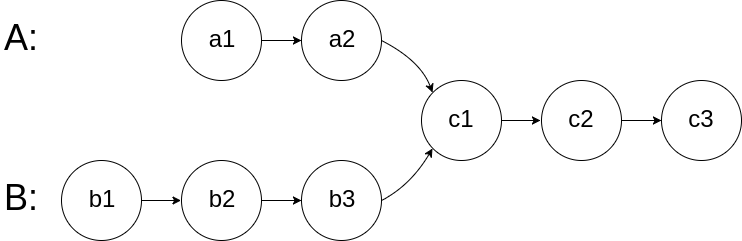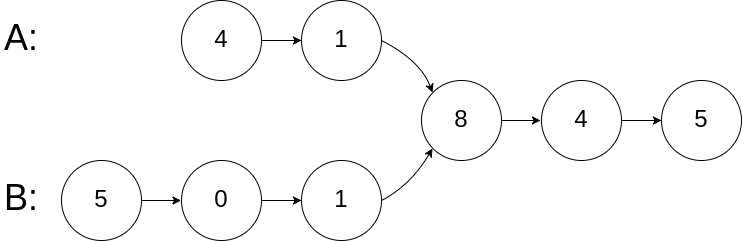输入：intersectVal = 8, listA = [4,1,8,4,5], listB = [5,0,1,8,4,5], skipA = 2, skipB = 3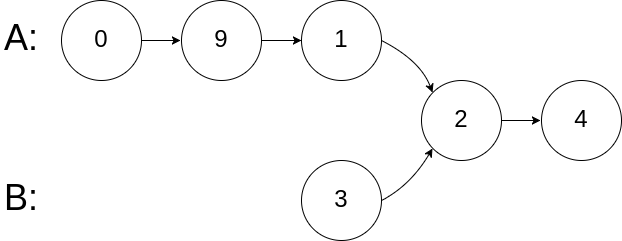输入：intersectVal = 2, listA = [0,9,1,2,4], listB = [3,2,4], skipA = 3, skipB = 1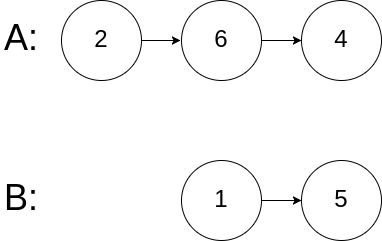输入：intersectVal = 0, listA = [2,6,4], listB = [1,5], skipA = 3, skipB = 2

• listA 中节点数目为 m
• listB 中节点数目为 n
• 0 <= m, n <= 3 * 104
• 1 <= Node.val <= 105
• 0 <= skipA <= m
• 0 <= skipB <= n
• 如果 listA 和 listB 没有交点，intersectVal 为 0
• 如果 listA 和 listB 有交点，intersectVal == listA[skipA + 1] == listB[skipB + 1]

pA走过的路径为A链+B链

pB走过的路径为B链+A链

pA和pB走过的长度都相同，都是A链和B链的长度之和，相当于将两条链从尾端对齐，如果相交，则会提前在相交点相遇，如果没有相交点，则会在最后相遇。

pA:1->2->3->4->5->6->null->9->5->6->null
pB:9->5->6->null->1->2->3->4->5->6->null
/**
* Definition for singly-linked list.
* struct ListNode {
*     int val;
*     ListNode *next;
*     ListNode(int x) : val(x), next(NULL) {}
* };
*/
class Solution {
public:
ListNode *p = headA, *q = headB;
while (p != q) {
p = p ? p->next : headB;
q = q ? q->next : headA;
}
return p;
}
};

## 【Day40】203.移除链表元素

#### 203. 移除链表元素输入：head = [1,2,6,3,4,5,6], val = 6

输入：head = [], val = 1

输入：head = [7,7,7,7], val = 7

• 列表中的节点在范围 [0, 104] 内
• 1 <= Node.val <= 50
• 0 <= k <= 50

/**
* Definition for singly-linked list.
* struct ListNode {
*     int val;
*     ListNode *next;
*     ListNode() : val(0), next(nullptr) {}
*     ListNode(int x) : val(x), next(nullptr) {}
*     ListNode(int x, ListNode *next) : val(x), next(next) {}
* };
*/
class Solution {
public:
ListNode *removeElements(ListNode *head, int val)
{
}
};

## 【Day41】474.一和零

#### 474. 一和零

输入：strs = ["10", "0001", "111001", "1", "0"], m = 5, n = 3

输入：strs = ["10", "0", "1"], m = 1, n = 1

• 1 <= strs.length <= 600
• 1 <= strs[i].length <= 100
• strs[i] 仅由 ‘0’ 和 ‘1’ 组成
• 1 <= m, n <= 100

const int N = 101;
class Solution {
public:
int dp[N][N];

int findMaxForm(vector<string>& strs, int m, int n)
{
for (auto &str:strs)
{
int one = 0, zero = 0;
for (auto &c:str)
{
if(c=='1')
++one;
else
++zero;
}
// zero = str.size() - one;
for (int j=m; j>=zero; --j)
{
for (int k=n; k>=one; --k)
{
dp[j][k] = max(dp[j][k], dp[j-zero][k-one] + 1);
}
}
}
return dp[m][n];
}
};

## 【Day42】494. 目标和

#### 494. 目标和

输入：nums = [1,1,1,1,1], target = 3

-1 + 1 + 1 + 1 + 1 = 3
+1 - 1 + 1 + 1 + 1 = 3
+1 + 1 - 1 + 1 + 1 = 3
+1 + 1 + 1 - 1 + 1 = 3
+1 + 1 + 1 + 1 - 1 = 3

输入：nums = , target = 1

• 1 <= nums.length <= 20
• 0 <= nums[i] <= 1000
• 0 <= sum(nums[i]) <= 1000
• -1000 <= target <= 100

class Solution {
int n;
int ans = 0;
int t;
public:
int findTargetSumWays(vector<int>& nums, int target) {
n = nums.size();
t = target;
dfs(0,nums,  0);
return ans;
}
void dfs(int u, vector <int> & nums, int s){
if(u == n){
if(s == t) ++ans;
return;
}
dfs(u + 1, nums, s + nums[u]);
dfs(u + 1, nums, s - nums[u]);
}
};

## 【Day43】1049. 最后一块石头的重量 II

#### 1049. 最后一块石头的重量 II

• 如果 x == y，那么两块石头都会被完全粉碎；
• 如果 x != y，那么重量为 x 的石头将会完全粉碎，而重量为 y 的石头新重量为 y-x。

输入：stones = [2,7,4,1,8,1]

输入：stones = [31,26,33,21,40]

输入：stones = [1,2]

• 1 <= stones.length <= 30

• 1 <= stones[i] <= 100

题解：

class Solution {
public:
int lastStoneWeightII(vector<int>& stones) {
auto sum = accumulate(stones.begin(), stones.end(), 0);
vector<int> dp(sum / 2 + 1);
for (const auto& stone : stones) {
for (auto j = sum / 2; j >= stone; j--) {
dp[j] = max(dp[j], dp[j - stone] + stone);
}
}
return sum - dp[sum / 2] * 2;
}
};


## 【Day44】879. 盈利计划

#### 879. 盈利计划

输入：n = 5, minProfit = 3, group = [2,2], profit = [2,3]

输入：n = 10, minProfit = 5, group = [2,3,5], profit = [6,7,8]

• 1 <= n <= 100
• 0 <= minProfit <= 100
• 1 <= group.length <= 100
• 1 <= group[i] <= 100
• profit.length == group.length
• 0 <= profit[i] <= 100

class Solution {
public:
int profitableSchemes(int n, int minProfit, vector<int>& group, vector<int>& profit) {
int mod = 1e9 + 7;
int size = group.size();
int dp[n + 1][minProfit + 1];
memset(dp, 0, sizeof(dp));
for (int i = 0; i <= n; ++i) {
dp[i] = 1;
}

for (int i = 1; i <= size; ++i) {
int cur_group = group[i - 1], cur_profit = profit[i - 1];
for (int j = n; j >= cur_group; --j) {
for (int k = minProfit; k >= 0; --k) {
dp[j][k] += dp[j - cur_group][max(k - cur_profit, 0)];
dp[j][k] %= mod;
}
}
}
return dp[n][minProfit];
}
};

## 【Day45】518. 零钱兑换 II

#### 518. 零钱兑换 II

输入: amount = 5, coins = [1, 2, 5]

5=5
5=2+2+1
5=2+1+1+1
5=1+1+1+1+1

输入: amount = 3, coins = 

输入: amount = 10, coins = 

• 0 <= amount (总金额) <= 5000
• 1 <= coin (硬币面额) <= 5000
• 硬币种类不超过 500 种
• 结果符合 32 位符号整数

class Solution {
public:
int change(int amount, vector<int>& coins) {
int n = coins.size();
vector<int> dp(amount+1);
dp = 1;
for(int i = 1; i<=n; i++)
{
int val = coins[i-1];
for(int j = val; j <= amount;++j)
{
dp[j] += dp[j-val];
}
}
return dp[amount];
}
};

## 【Day46】279. 完全平方数

#### 279. 完全平方数

输入：n = 12

输入：n = 13

• 1 <= n <= 104

//假设最小公式值m = ƒ(n)
//那么n的值满足下列公式 ∑(A[i] * A[i]) = n
//令 k 为满足最小值 m 的时候，最大的平方数  。 令  d + k * k; = n ;  d >= 0;
// 注意：一定要是满足m最小的时候的k值,一味的取最大平方数,就是贪心算法了
//得出 f(d) + f(k*k) = f(n);
//显然 f(k*k) = 1; 则  f(d) + 1 = f(n); 因为 d = n - k*k;
//则可以推出ƒ(n - k * k) + 1 = ƒ(n) ;  且 k * k <= n;

class Solution {
public:
int numSquares(int n) {
if (n <= 0) return 0;
std::vector<int> nums(n+1);
for(int i = 1; i <= n;i++)
{
int min = i;
for(int j = 1;i - j*j >= 0;j++)
{
int tmp = nums[i-j*j] + 1;
min = min > tmp ?  tmp:min;
}
nums[i] = min;
}
return nums[n];

}
};

## 【Day47】1449. 数位成本和为目标值的最大数字

#### 1449. 数位成本和为目标值的最大数字

• 给当前结果添加一个数位（i + 1）的成本为 cost[i] （cost 数组下标从 0 开始）。
• 总成本必须恰好等于 target 。
• 添加的数位中没有数字 0 。

输入：cost = [4,3,2,5,6,7,2,5,5], target = 9

数字     成本
1  ->   4
2  ->   3
3  ->   2
4  ->   5
5  ->   6
6  ->   7
7  ->   2
8  ->   5
9  ->   5

输入：cost = [7,6,5,5,5,6,8,7,8], target = 12

输入：cost = [2,4,6,2,4,6,4,4,4], target = 5

输入：cost = [6,10,15,40,40,40,40,40,40], target = 47

• cost.length == 9
• 1 <= cost[i] <= 5000
• 1 <= target <= 5000

class Solution {
public:
string largestNumber(vector<int>& cost, int target) {
int len=cost.size();
vector<string> dp(target+1,"#");
dp="";
//因为i遍历的顺序是从小到大，就保证了整数的高位一定大于等于整数的低位，也就保证了整数最大
for(int i=1;i<len+1;++i)
for(int j=1;j<target+1;++j){
if(j>=cost[i-1]&&dp[j-cost[i-1]]!="#"){
dp[j]=CompareString(dp[j],to_string(i)+dp[j-cost[i-1]]);
}
}
return dp[target]=="#"?"0":dp[target];
}
private:
string CompareString(const string& s1,const string& s2){
int m=s1.size(),n=s2.size();
if(m==n)return max(s1,s2);
return m>n?s1:s2;
}
};

## 【Day48】278. 第一个错误的版本

#### 278. 第一个错误的版本

给定 n = 5，并且 version = 4 是第一个错误的版本。

bool isBadVersion(int version);

class Solution {
public:
int firstBadVersion(int n) {
int lo = 1;
int hi = n;

while(lo < hi) {
int mid = lo + (hi - lo) / 2;
hi = mid;
} else {
lo = mid + 1;
}
}
return hi;
}
};

## 【Day49】374. 猜数字大小

#### 374. 猜数字大小

• 每轮游戏，我都会从 1 到 n 随机选择一个数字。 请你猜选出的是哪个数字。
• 如果你猜错了，我会告诉你，你猜测的数字比我选出的数字是大了还是小了。

• -1：我选出的数字比你猜的数字小 pick < num
• 1：我选出的数字比你猜的数字大 pick > num
• 0：我选出的数字和你猜的数字一样。恭喜！你猜对了！pick == num

输入：n = 10, pick = 6

输入：n = 1, pick = 1

输入：n = 2, pick = 1

输入：n = 2, pick = 2

• 1 <= n <= 231 - 1
• 1 <= pick <= n

/**
* Forward declaration of guess API.
* @param  num   your guess
* @return          -1 if num is lower than the guess number
*                  1 if num is higher than the guess number
*               otherwise return 0
* int guess(int num);
*/

class Solution {
public:
int guessNumber(int n) {
int l = 0;
int r = n;
while (l <= r)
{
int m = (l-r)/2 + r;
int res = guess(m);
if (res == 0)
{
return m;
}
else if (res < 0)
{
r = m-1;
}
else
{
l = m + 1;
}
}

return -1;
}
};

## 【Day50】852. 山脉数组的峰顶索引

#### 852. 山脉数组的峰顶索引

• arr.length >= 3

• 存在 i（0 < i < arr.length - 1）使得：

• arr < arr < … arr[i-1] < arr[i]
• arr[i] > arr[i+1] > … > arr[arr.length - 1]

给你由整数组成的山脉数组 arr ，返回任何满足 arr < arr < … arr[i - 1] < arr[i] > arr[i + 1] > … > arr[arr.length - 1] 的下标 i 。

输入：arr = [0,1,0]

输入：arr = [0,2,1,0]

输入：arr = [0,10,5,2]

输入：arr = [3,4,5,1]

输入：arr = [24,69,100,99,79,78,67,36,26,19]

• 3 <= arr.length <= 104
• 0 <= arr[i] <= 106
• 题目数据保证 arr 是一个山脉数组

class Solution {
public:
int peakIndexInMountainArray(vector<int>& arr) {
//根据 arr[mid-1]和arr[mid]对比
//所以搜索范围是 [1,arr.length -1]
int left = 1;
int right = arr.size() - 1;

while(left < right){
//如果check 函数调整的是left，则+1. 否则有可能陷入死循环
int mid = left + right + 1 >> 1;
if(arr[mid-1] < arr[mid])
left = mid;
else
right = mid -1;
}
return right;
}
};

## 【Day51】877. 石子游戏

#### 877. 石子游戏

输入：[5,3,4,5]

• 2 <= piles.length <= 500
• piles.length 是偶数。
• 1 <= piles[i] <= 500
• sum(piles) 是奇数。

class Solution {
public:
bool stoneGame(vector<int>& piles) {
return true;
}
};

## 【Day52】65. 有效数字

1. 一个 小数 或者 整数
2. （可选）一个 ‘e’ 或 ‘E’ ，后面跟着一个 整数

1. （可选）一个符号字符（’+’ 或 ‘-‘）
2. 下述格式之一：
1. 至少一位数字，后面跟着一个点 ‘.’
2. 至少一位数字，后面跟着一个点 ‘.’ ，后面再跟着至少一位数字
3. 一个点 ‘.’ ，后面跟着至少一位数字

• （可选）一个符号字符（’+’ 或 ‘-‘）
• 至少一位数字

• [“2”, “0089”, “-0.1”, “+3.14”, “4.”, “-.9”, “2e10”, “-90E3”, “3e+7”, “+6e-1”, “53.5e93”, “-123.456e789”]

• [“abc”, “1a”, “1e”, “e3”, “99e2.5”, “–6”, “-+3”, “95a54e53”]

输入：s = "0"

输入：s = "e"

输入：s = "."

输入：s = ".1"

• 1 <= s.length <= 20
• s 仅含英文字母（大写和小写），数字（0-9），加号 ‘+’ ，减号 ‘-‘ ，或者点 ‘.’ 。

class Solution {
public:
bool isNumber(string s) {
int l = 0, r = s.size() - 1;
//去掉前后空格
while (l <= r && s[l] == ' ') l ++ ;
while (l <= r && s[r] == ' ') r -- ;
if (l > r) return false;
s = s.substr(l, r - l + 1);
//去掉正负号
if (s == '+' || s == '-') s = s.substr(1);
//如果只有正负号，false
if (s.empty()) return false;
//如果只有一个点，或者.e || .E 返回false
if (s == '.' && (s.size() == 1 || s == 'e' || s == 'E'))
return false;

//记录点和e的次数
int dot = 0, e = 0;
for (int i = 0; i < s.size(); i ++ ) {
if (s[i] == '.') {
//如果出现多个点或者e ，fasle
if (dot > 0 || e > 0) return false;
dot ++ ;
} else if (s[i] == 'e' || s[i] == 'E') {
//如果e的前面没东西或者后面没东西，或者多个e ，false
if (!i || i + 1 == s.size() || e > 0) return false;
//如果e的后面是正负号
if (s[i + 1] == '+' || s[i + 1] == '-') {
//但是后面没东西了，返回false
if (i + 2 == s.size()) return false;
i ++ ;
}
e ++ ;
//不合法
} else if (s[i] < '0' || s[i] > '9') return false;
}
return true;
}
};

## 【Day53】483. 最小好进制

#### 483. 最小好进制

输入："13"

输入："4681"

输入："1000000000000000000"

1. n的取值范围是 [3, 10^18]。
2. 输入总是有效且没有前导 0。

class Solution {
public:
using LL = long long;
string smallestGoodBase(string n) {
LL x = stoll(n);
for(int m = 2; m <= 60; m++){
LL k = (LL)pow(x, 1. / m);
if(k > 1){
LL cur = 1;
for(LL i = 1, base = 1; i <= m; i++){
base *= k;
cur += base;
}
}
}
}
};

## 【Day54】1239. 串联字符串的最大长度

#### 1239. 串联字符串的最大长度

输入：arr = ["un","iq","ue"]

输入：arr = ["cha","r","act","ers"]

输入：arr = ["abcdefghijklmnopqrstuvwxyz"]

• 1 <= arr.length <= 16
• 1 <= arr[i].length <= 26
• arr[i] 中只含有小写英文字母

class Solution {
public:
int maxLength(vector<string> &arr) {
int ans = 0;
vector<int> masks = {0};
for (string &s : arr) {
int mask = 0;
for (char ch : s) {
ch -= 'a';
if ((mask >> ch) & 1) { // 若 mask 已有 ch，则说明 s 含有重复字母，无法构成可行解
break;
}
mask |= 1 << ch; // 将 ch 加入 mask 中
}
if (mask == 0) {
continue;
}
int n = masks.size();
for (int i = 0; i < n; ++i) {
int m = masks[i];
if ((m & mask) == 0) { // m 和 mask 无公共元素
ans = max(ans, __builtin_popcount(m | mask));
}
}
}
return ans;
}
};

## 【Day55】1600.皇位继承顺序

#### 1600. 皇位继承顺序

Successor(x, curOrder):

1. 一开始， curOrder 为 [“king”].
2. 调用 Successor(king, curOrder) ，返回 Alice ，所以我们将 Alice 放入 curOrder 中，得到 [“king”, “Alice”] 。
3. 调用 Successor(Alice, curOrder) ，返回 Jack ，所以我们将 Jack 放入 curOrder 中，得到 [“king”, “Alice”, “Jack”] 。
4. 调用 Successor(Jack, curOrder) ，返回 Bob ，所以我们将 Bob 放入 curOrder 中，得到 [“king”, “Alice”, “Jack”, “Bob”] 。
5. 调用 Successor(Bob, curOrder) ，返回 null 。最终得到继承顺序为 [“king”, “Alice”, “Jack”, “Bob”] 。

• ThroneInheritance(string kingName) 初始化一个 ThroneInheritance 类的对象。国王的名字作为构造函数的参数传入。
• void birth(string parentName, string childName) 表示 parentName 新拥有了一个名为 childName 的孩子。
• void death(string name) 表示名为 name 的人死亡。一个人的死亡不会影响 Successor 函数，也不会影响当前的继承顺序。你可以只将这个人标记为死亡状态。
• string[] getInheritanceOrder() 返回 除去 死亡人员的当前继承顺序列表。

["ThroneInheritance", "birth", "birth", "birth", "birth", "birth", "birth", "getInheritanceOrder", "death", "getInheritanceOrder"]
[["king"], ["king", "andy"], ["king", "bob"], ["king", "catherine"], ["andy", "matthew"], ["bob", "alex"], ["bob", "asha"], [null], ["bob"], [null]]

[null, null, null, null, null, null, null, ["king", "andy", "matthew", "bob", "alex", "asha", "catherine"], null, ["king", "andy", "matthew", "alex", "asha", "catherine"]]

ThroneInheritance t= new ThroneInheritance("king"); // 继承顺序：king
t.birth("king", "andy"); // 继承顺序：king > andy
t.birth("king", "bob"); // 继承顺序：king > andy > bob
t.birth("king", "catherine"); // 继承顺序：king > andy > bob > catherine
t.birth("andy", "matthew"); // 继承顺序：king > andy > matthew > bob > catherine
t.birth("bob", "alex"); // 继承顺序：king > andy > matthew > bob > alex > catherine
t.birth("bob", "asha"); // 继承顺序：king > andy > matthew > bob > alex > asha > catherine
t.getInheritanceOrder(); // 返回 ["king", "andy", "matthew", "bob", "alex", "asha", "catherine"]
t.death("bob"); // 继承顺序：king > andy > matthew > bob（已经去世）> alex > asha > catherine
t.getInheritanceOrder(); // 返回 ["king", "andy", "matthew", "alex", "asha", "catherine"]

1. 1 <= kingName.length, parentName.length, childName.length, name.length <= 15
2. kingName，parentName， childName 和 name 仅包含小写英文字母。
3. 所有的参数 childName 和 kingName 互不相同。
4. 所有 death 函数中的死亡名字 name 要么是国王，要么是已经出生了的人员名字。
5. 每次调用 birth(parentName, childName) 时，测试用例都保证 parentName 对应的人员是活着的。
6. 最多调用 105 次birth 和 death 。
7. 最多调用 10 次 getInheritanceOrder 。

class ThroneInheritance {
public:
string root;
unordered_map<string, bool> isdeath;
unordered_map<string, vector<string>> family;
ThroneInheritance(string kingName) {
root = kingName;
}

void birth(string parentName, string childName) {
family[parentName].push_back(childName);
}

void death(string name) {
isdeath[name] = true;
}

void dfs(vector<string>& ans, string root) {
if (!isdeath[root])
ans.push_back(root);
for (string str: family[root])
dfs(ans, str);
}

vector<string> getInheritanceOrder() {
vector<string> ans;
dfs(ans, root);
return ans;
}
};

/**
* Your ThroneInheritance object will be instantiated and called as such:
* ThroneInheritance* obj = new ThroneInheritance(kingName);
* obj->birth(parentName,childName);
* obj->death(name);
* vector<string> param_3 = obj->getInheritanceOrder();
*/

## 【Day56】401.二进制手表

#### 401. 二进制手表

• 例如，下面的二进制手表读取 “3:25” 。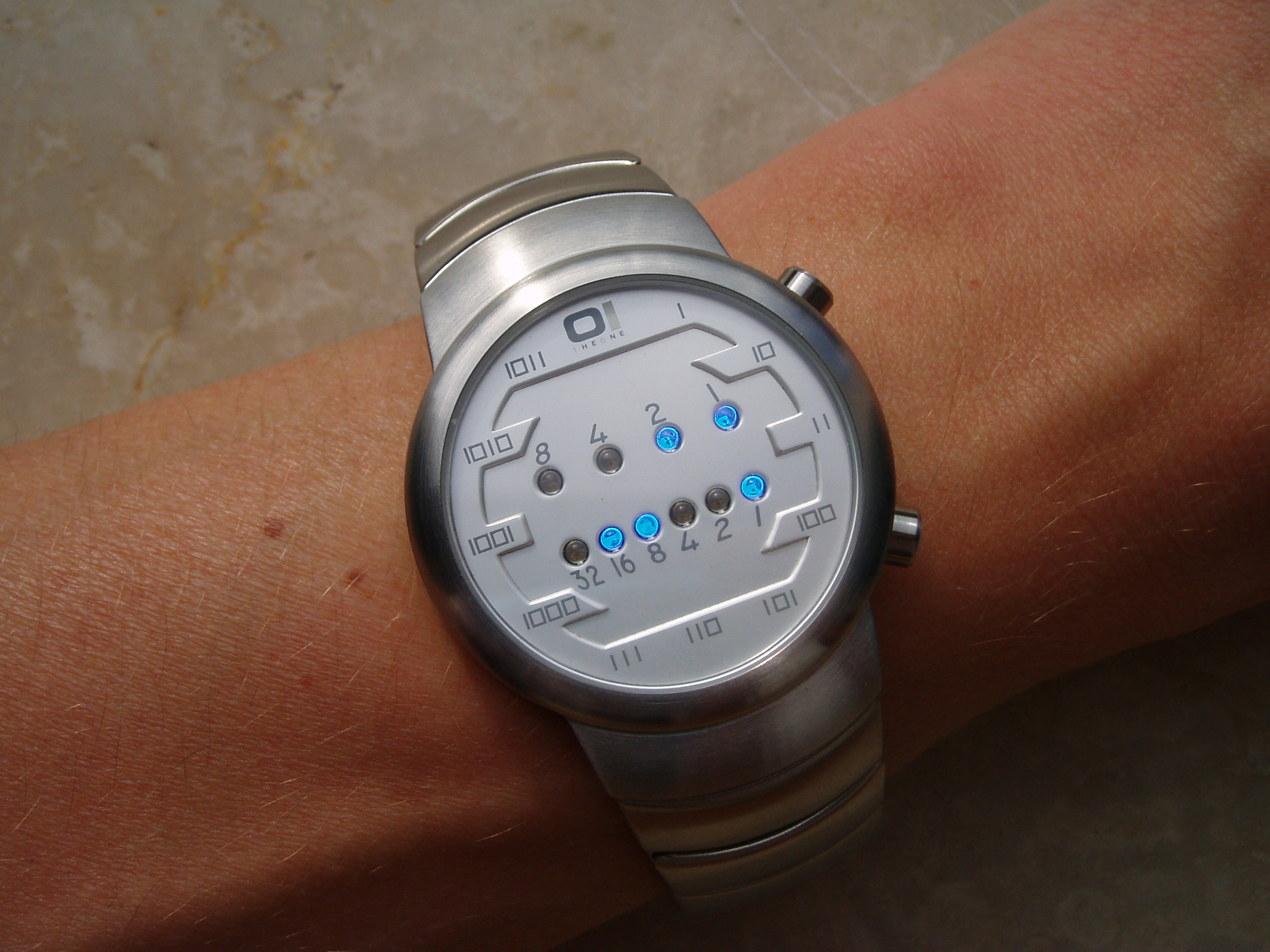• 例如，”01:00” 是无效的时间，正确的写法应该是 “1:00” 。

输入：turnedOn = 1

输入：turnedOn = 9

• 0 <= turnedOn <= 10

class Solution {
public:
vector<string> readBinaryWatch(int num) {
vector<string> res;
//直接遍历  0:00 -> 12:00   每个时间有多少1
for (int i = 0; i < 12; i++) {
for (int j = 0; j < 60; j++) {
if (count1(i) + count1(j) == num) {
res.push_back(to_string(i)+":"+
(j < 10 ? "0"+to_string(j) : to_string(j)));
}
}
}
return res;
}
//计算二进制中1的个数
int count1(int n) {
int res = 0;
while (n != 0) {
n = n & (n - 1);
res++;
}
return res;
}
};



## 【Day57】剑指 Offer 15.二进制中1的个数

#### 剑指 Offer 15. 二进制中1的个数

输入：00000000000000000000000000001011

输入：00000000000000000000000010000000

输入：11111111111111111111111111111101

• 输入必须是长度为 32 的 二进制串 。

class Solution {
public:
int hammingWeight(uint32_t n) {
int ans = 0;
for (int i = 0; i < 32; i++) {
ans += ((n >> i) & 1);
}
return ans;
}
};

## 【Day58】149. 直线上最多的点数

#### 149. 直线上最多的点数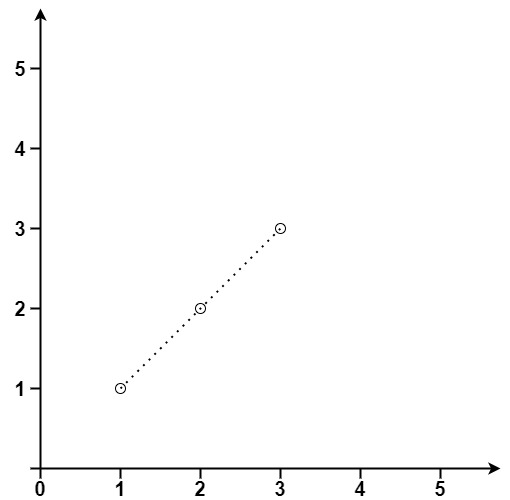输入：points = [[1,1],[2,2],[3,3]]输入：points = [[1,1],[3,2],[5,3],[4,1],[2,3],[1,4]]

• 1 <= points.length <= 300
• points[i].length == 2
• -104 <= xi, yi <= 104
• points 中的所有点 互不相同

class Solution {
public:
int maxPoints(vector<vector<int>>& points) {
int len = points.size();
// 点的数量不够
if(len < 3) {
return len;
}
int maxNum = 2;
// 遍历每两个点
for(int i = 0; i < len - 1; i ++) {
for(int j = i + 1; j < len; j ++) {
// 统计斜率相等个数
int count = 2;
long long dx = points[i] - points[j];
long long dy = points[i] - points[j];
// 与其他点比较
for(int k = j + 1; k < len; k ++) {
// 如果斜率相等
if(dx * (points[i] - points[k]) == dy * (points[i] - points[k])) {
count ++;
}
}
maxNum = max(maxNum, count);
if(maxNum > len / 2) return maxNum;
}
}
return maxNum;
}
};

## 【Day59】752.打开转盘锁

#### 752. 打开转盘锁

输入：deadends = ["0201","0101","0102","1212","2002"], target = "0202"

输入: deadends = ["8888"], target = "0009"

输入: deadends = ["8887","8889","8878","8898","8788","8988","7888","9888"], target = "8888"

输入: deadends = ["0000"], target = "8888"

• 1 <= deadends.length <= 500
• deadends[i].length == 4
• target.length == 4
• target 不在 deadends 之中
• target 和 deadends[i] 仅由若干位数字组成

class Solution {
public:
int seen;//记录搜到过的轮盘锁数字
int ans = -1;
int q;//模拟队列
int openLock(vector<string>& deadends, string target) {
for(auto d : deadends){

int tmp = stoi(d);
}

//如果"0000"是死亡数字直接返回0,如果target为0000直接返回-1
if(target == "0000") return 0;
int tar=stoi(target);
bfs(tar);
return ans;
}

{
if(num == 10)
return -9;
if(num == -1)
return -9;
else return 1;
}

void bfs(int target)
{
//hh队头，tt队尾
int hh=0,tt=0;

//先将队头初始化为0,代表从"0000"开始旋转
q = 0;
while(hh <= tt){
auto t = q[hh++];
int prev = t;

for(int i = 1 ; i <= 1000 ; i *= 10){
int num = t / i % 10;
int temp=t;

//正向旋转当前位的数字
int tmp = temp + numsadd(num+1)*i;

//满足条件就将temp插入队尾
q[++tt] = tmp;
//更新新转盘锁数字的操作次数
seen[tmp] = seen[prev]+1;
}

//反向旋转当前位的数字
tmp = temp - numsadd(num-1)*i;
q[++tt] = tmp;
seen[tmp] = seen[prev]+1;
}
}

if(seen[target] != 0){
ans = seen[target];
break;
}
}
}
};

转载规则

《暑假LeetCode刷题集合（下）》 Tyzhao 采用 知识共享署名 4.0 国际许可协议 进行许可。
目录